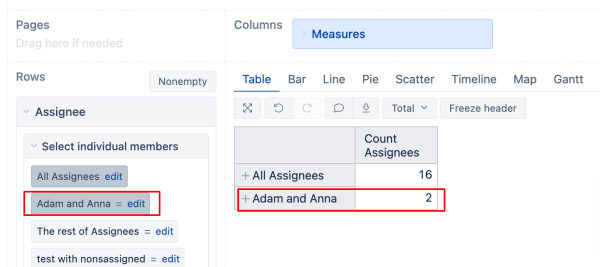# Count for Aggregate function

Hi,

I’m trying to count number of items in Aggregate({ }) function?
How to get Count in new Define calculated member formula?
I have in Logged By
Aggregate({
[Logged by].[name1],
[Logged by].[name2],
[Logged by].[name3],
[Logged by].[name4]
})
And in calculated member formula i’m trying to get [Measures].[Logged By].Count
But it returns 1, not 4. How it can be done, please advise.

Thank you

Hi @Tatyana_Terehova

​I recommend creating a new calculated measure with the formula below to count all the members.

`​ChildrenSet([Logged By].[Logged By]).Count`

​Below is an example of the Assignee dimension, where I have a calculated member with two users, Adam and Anna.
​When using the above formula, it returns value 2, which is exactly how many users are aggregated in the calculated member.Best wishes,

Elita from support@eazybi.com

1 Like

Hi @Elita.Kalane ,
Thank you so much, that’s exactly what I was trying to do. It helped me a lot!

1 Like